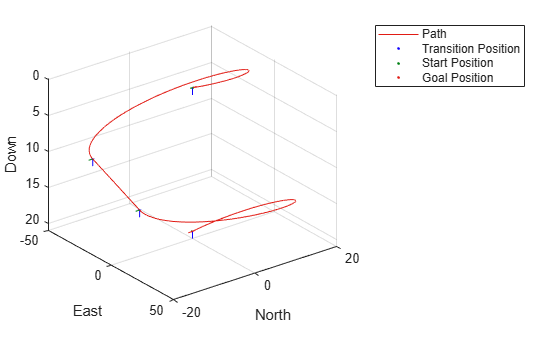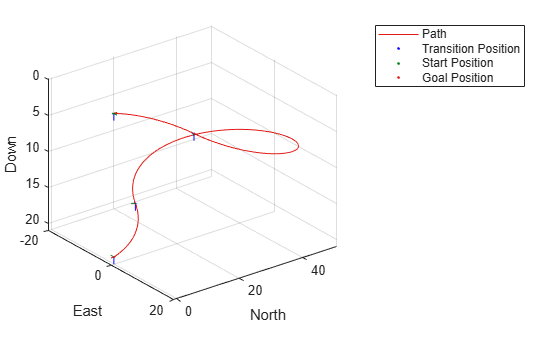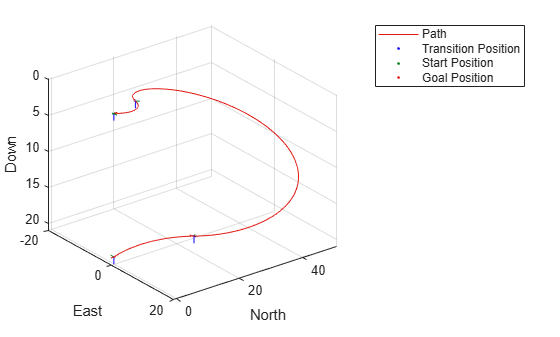connect

Connect poses with UAV Dubins connection path

Description

[pathSegObj,pathCost] = connect(connectionObj,start,goal) connects the start and goal poses using the specified uavDubinsConnection object. The path segment object with the lowest cost is returned.

example

[pathSegObj,pathCost] = connect(connectionObj,start,goal,'PathSegments','all') returns all possible path segments as a cell array with their associated costs.

Examples

collapse all

This example shows how to calculate all valid UAV Dubins path segments and connect poses using the uavDubinsConnection object.

Calculate All Possible Path Segments

Create a uavDubinsConnection object.

connectionObj = uavDubinsConnection;

Define start and goal poses as [x, y, z, headingAngle] vectors.

startPose = [0 0 0 0]; % [meters, meters, meters, radians]
goalPose = [0 0 20 pi];

Calculate all possible path segments and connect the poses.

[pathSegObj,pathCosts] = connect(connectionObj,startPose,goalPose,'PathSegments','all');

Path Validation and Visualization

Check the validity of all the possible path segments and display the valid paths along with their motion type and path cost.

for i = 1:length(pathSegObj)
if ~isnan(pathSegObj{i}.Length)
figure
show(pathSegObj{i})
fprintf('Motion Type: %s\nPath Cost: %f\n',strjoin(pathSegObj{i}.MotionTypes),pathCosts(i));
end
endMotion Type: L S L N
Path Cost: 214.332271Motion Type: R S R N
Path Cost: 214.332271Motion Type: R L R N
Path Cost: 138.373157Motion Type: L R L N
Path Cost: 138.373157

Input Arguments

collapse all

Path connection type, specified as a uavDubinsConnection object. This object defines the parameters of the connection.

Initial pose of the UAV at the start of the path segment, specified as a four-element numeric vector or matrix [x, y, z, headingAngle].

x, y, and z specify the position in meters. headingAngle specifies the heading angle in radians. The heading angle is measured clockwise from north to east. Each row of the matrix corresponds to a different start pose.

The pose follows the north-east-down coordinate system.

The start and goal pose inputs can be any of these combinations:

• Single start pose with single goal pose.

• Multiple start poses with single goal pose.

• Single start pose with multiple goal poses.

• Multiple start poses with multiple goal poses.

Goal pose of the UAV at the end of the path segment, specified as a four-element numeric vector or matrix [x, y, z, headingAngle].

x, y, and z specify the position in meters. headingAngle specifies the heading angle in radians. The heading angle is measured clockwise from north to east. Each row of the matrix corresponds to a different goal pose.

The pose follows the north-east-down coordinate system.

The start and goal pose inputs can be any of these combinations:

• Single start pose with single goal pose.

• Multiple start poses with single goal pose.

• Single start pose with multiple goal poses.

• Multiple start poses with multiple goal poses.

Output Arguments

collapse all

Path segments, returned as a cell array of uavDubinsPathSegment objects. The type of object depends on the input connectionObj. The size of the cell array depends on whether you use single or multiple start and goal poses.

By default, the function returns the path with the lowest cost for each start and goal pose.

When calling the connect function using the 'PathSegments','all' name-value pair, the cell array contains all valid path segments between the specified start and goal poses.

Cost of path segments, returned either as a positive numeric scalar, vector, or matrix. Each element of the cost vector corresponds to a path segment in pathSegObj.

By default, the function returns the path with the lowest cost for each start and goal pose.

Extended Capabilities

C/C++ Code GenerationGenerate C and C++ code using MATLAB® Coder™.